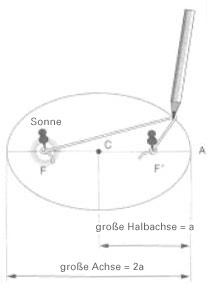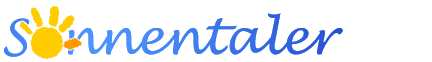# Why are the orbits of planets elliptical

## The orbits are always elliptical

 Author: Publication: 1.9.2000 Origin: La main à la pâte, Paris

We have already seen that a planet's orbit is an ellipse. Johannes Kepler (1571-1630) was the first to establish this thanks to the great accuracy of the observations made by his teacher Tycho Brahe (1546-1601). Kepler formulated the first of the laws of the solar system named after him as follows:

"The planets describe orbits around the sun that have the shape of ellipses. The sun is not in the center of the ellipse, but to the side at a point called the" focal point "."

By definition, an ellipse is "the locus of all points for which the sum of the distances from two fixed points (focal points) is a constant". For illustration, we recommend using pencil and thread to draw an ellipse on paper using the "gardening method".Draw an ellipse using the gardening method

It's very simple: you press two thumbtacks into the paper at points F and F '. This defines the two focal points of the ellipse. Now you connect the two points with a thread that is longer than the distance FF '. The thread is held taut with a pencil, which is drawn over the paper around the two focal points. The result is an ellipse. It can be seen that the two focal points lie on the major axis of the ellipse (big axis) at the same distance from the center C, and that the length of the major axis (= 2a) is equal to the length of the thread.

An ellipse can be more or less flattened, depending on the distance between the two focal points (the circle is a special case of the ellipse, the two focal points then coincide). The degree of flattening is given by a parameter: The eccentricity (which we denote by "e") is the ratio of the distance between the two focal points and the length of the major axis: e = FF '/ 2a (e = 0 applies to the circle ; the closer the eccentricity of a planet's orbit approaches the value 0, the more circular the orbit). In the solar system the eccentricities are relatively small. Pluto has one of the most eccentric orbits (e = 0.249) in the solar system, as does the dwarf planet Eris (e = 0.44), while the earth's orbit is almost circular with e = 0.01675.

The above schematic illustration shows that the distance between the pen and the thumbtack, which represents the sun, is not constant. When a planet is closest to the Sun, it is called the perihelion of its orbit, the greatest distance from the Sun is called the aphelion. In the case of the earth's orbit, the two focal points are 4 million km apart and the earth-sun distance varies between approximately 147 million km (perihelion) and 151 million km (aphelion). The distance of the planets from the sun (shown here by one of the thumbtacks) varies during the orbit.

Attention: The inclination of the earth's axis of rotation against its orbit is responsible for the seasons and not the (far too weak) change in the earth-sun distance.

Last update: 11.2.2014

© Sonnentaler / La main à la pâte http://www.sonnentaler.net/